Trapezoid or Trapezium? and the Median.

Topic:
Trapezoid
A Trapezoid is an irregular quadrilateral with 4 unequal sides: 2 opposite parallel bases, 2 opposite non-parallel legs, 4 interior angles that equal 360 degrees, and an altitude (height) line that is the distant from one base line to the other base line at an right angle. ( Fun fact: Parallel are 2 lines of equal distant that never touch; perpendicular lines touch/intersect and form a 90 degree angle/right angle.)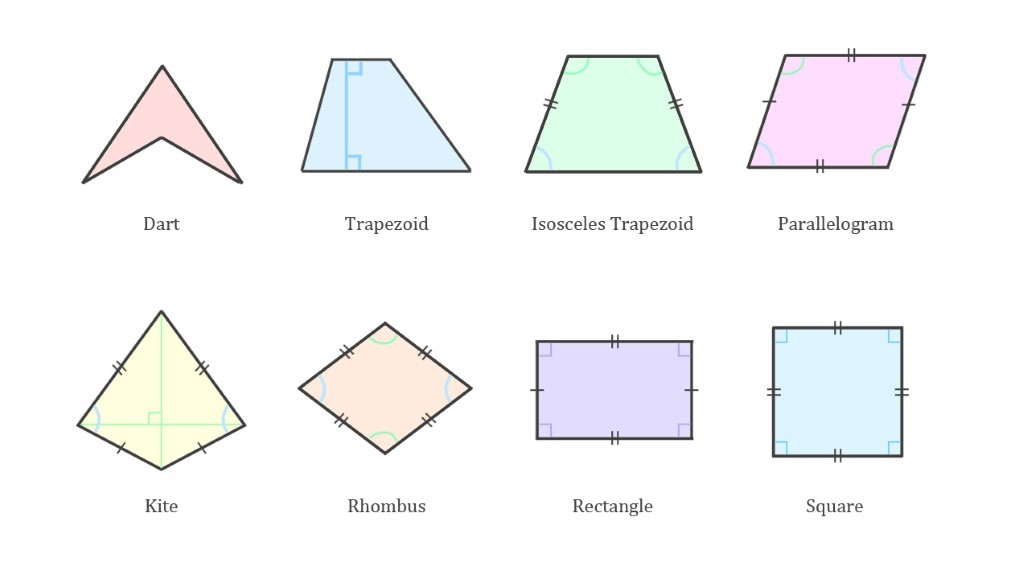2. Answer the trivial math fact?

There can be more than one altitude/height line within a trapezoid as long as the lines form a 90 degree angle. The altitude line is used to find the area of a trapezoid.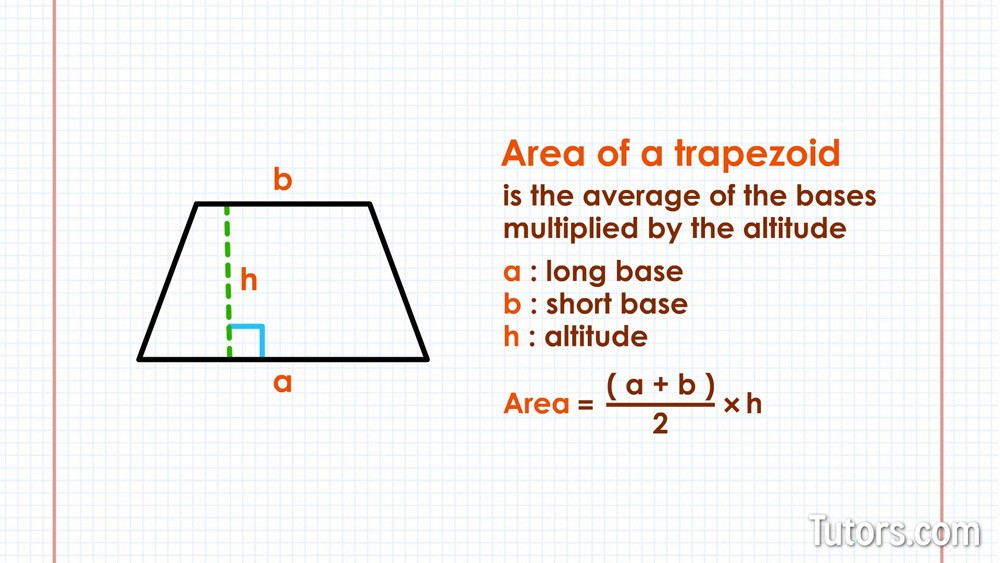A regular/scalene and irregular/isosceles trapezoid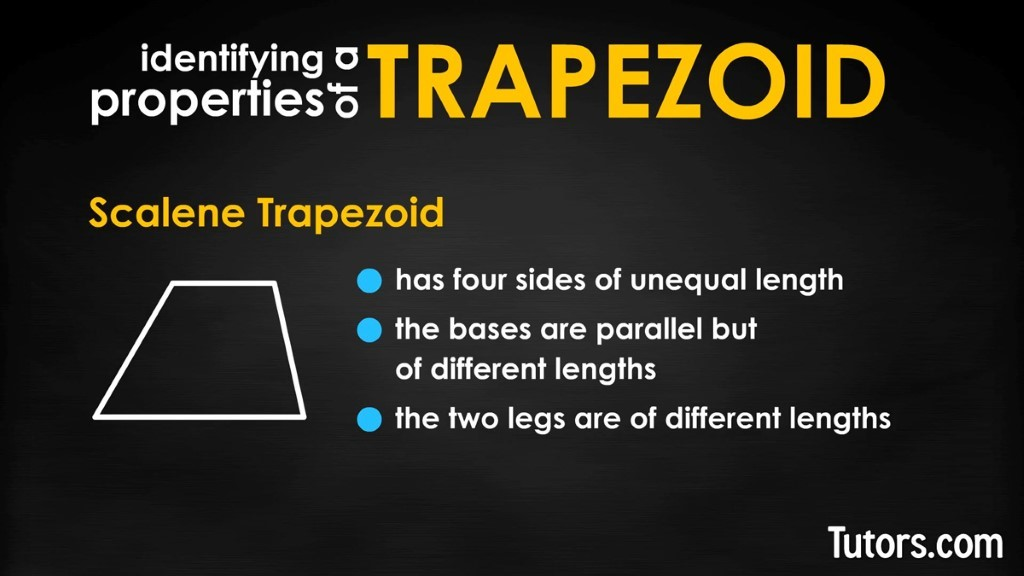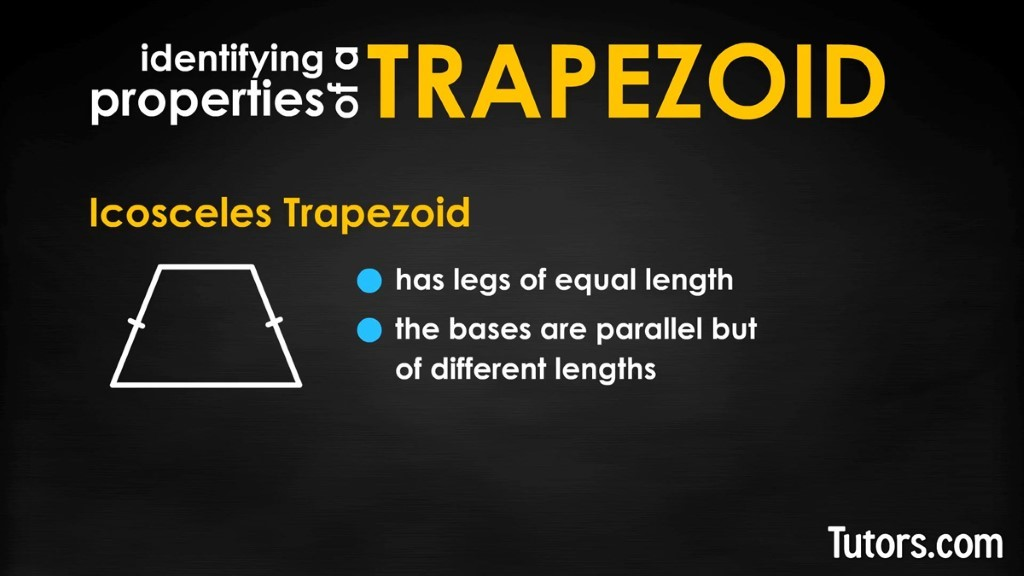Median of Trapezoid The Median (midline or midsegment) is a line that is half-way between the two bases. The median is the average of the 2 base lengths.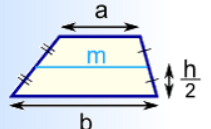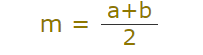Perimeter of Trapezoid The Perimeter is the sum of all 4 side lengths.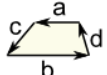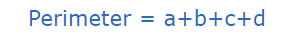4. Find the median, the area , and the perimeter.

1. b = 6 , b = 4, h = 3 2. b = 4, b = 2, h = 1.3 3. b = 3, b = 2, h = 1.9 4. b = 7, b = 6, h = 2

Create........

1. Using the numbers in question 4, create your own trapezoids; label them with the bases, legs, parallel lines, diagonals, and the altitude,